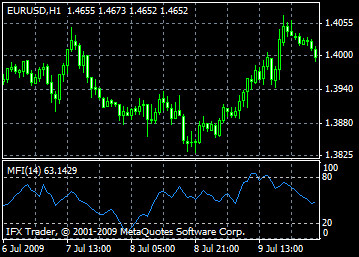Money Flow Index Indicator (MFI)

# Money Flow Index Indicator (MFI)

Technical Indicator Money Flow Index, MFI indicates the intensity of the money investments into the security. Construction and interpretation is similar to the Relative Strength Index, the only difference is that the MFI takes into account volume.

Analyzing Money Flow Index keep in mind the following:

Divergences between the indicator and price movement. If prices grow and Money Flow Index falls (or vice versa) there is a great possibility of the prices reverse;

Money Flow Index value above 80 and below 20 warns about the potential top and bottom of the market.#### Calculation

The calculation of Money Flow Index includes several stages. At first one defines the typical price (TP) of the period in question.

TP = (HIGH + LOW + CLOSE)/3

Then one calculates the amount of the Money Flow (MF):

MF = TP * VOLUME

If today`s typical price is larger than yesterday`s TP, then the money flow is considered positive. If today`s typical price is lower than that of yesterday, the money flow is considered negative.

A positive money flow is a sum of positive money flows for a selected period of time. A negative money flow is the sum of negative money flows for a selected period of time.

Then one calculates the money ratio (MR) by dividing the positive money flow by the negative money flow:

MR = Positive Money Flow (PMF)/Negative Money Flow (NMF)

And finally, one calculates the money flow index using the money ratio:

MFI = 100 - (100 / (1 + MR))

Take the first step towards your goal
Open account